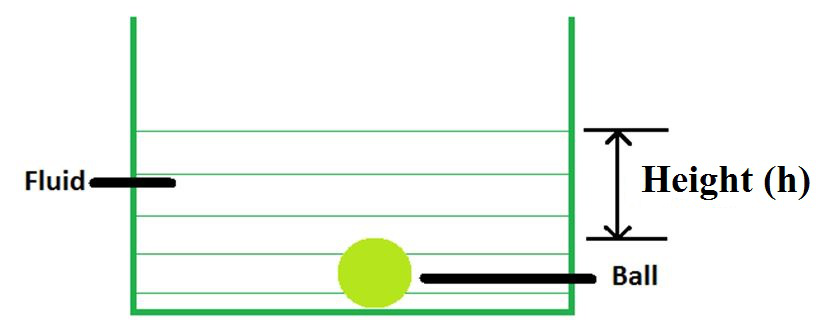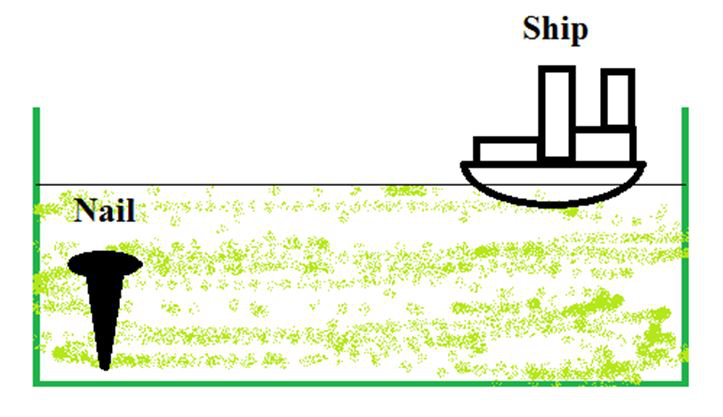GFG App
Open AppBrowser
Continue

Hydrostatic pressure can be stated as the pressure exerted by a fluid at equilibrium at a given point within the fluid, due to the force of gravity. Hydrostatic pressure is proportional to the depth measured from the surface as the weight of the fluid increases with a downward force applied.

The fluid pressure can be caused by gravity, acceleration, or forces when in a closed container. Consider a container filled with water or any other fluid along with a ball in the bottom. Now consider the ball from the top of the container. There is pressure exerted by the layer of water acting on the ball. As we move down from the top of the container to the bottom, the pressure exerted by the top layer on the ball adds up. This phenomenon is responsible for more pressure at the bottom of the container.As we can see in the above figure a ball is completely submerged in a fluid. Here force applied by the fluid on the ball equals to Hydrostatic pressure. Top Layer of the fluid exerts the most pressure on the ball. When we move the ball upside, the pressure applied by the top layer starts to reduce.

### Fluid pressure

Fluid pressure can be defined as the pressure on a given object on the surface of a closed container or in the fluid. Gravity, acceleration, or forces outside the container, are the factors that cause the pressure.

Pressure is a scalar quantity that is stated as force per unit area. Here force acts in the direction perpendicular to the surface. Depending on the context of use, there are a number of units in which pressure can be measured.

Pressure = Force/ Area

P = F / A

Where

P = Pressure

F = Force

A = Area

Unit of pressure is N/m2 or Pa (Pascal).

### Formulae of Hydrostatic PressureLet us derive the formula for Pressure on an object submerged in a fluid.

We know that,

Pressure = Force/Area = F/A & Force = mass x acceleration = m × g (acceleration due to gravity)

So: Pressure = F/A = mg/A ……………. (1)

Now Density = Mass/Volume;

So, Mass= Density x Volume ……………. (2)

From equation 1 & 2,

Pressure = (density x volume x acceleration)/area.

P = (ρ * g * v)/A

P = ρ * g * h

Here

h = v/A = Height of fluid above the object

ρ (rho) is the density of the fluid,

g is the acceleration of gravity

Pressure on an object submerged in a fluid is-

P = ρ * g * h

### Sample Problems

Problem 1. A scuba driver is diving 10 meters below the surface of the ocean. Find the pressure on the driver assuming the standard atmospheric conditions. Use the density of sea water = 1.03 X 103 kg/m3 and the atmospheric pressure of 1.01 x 105 N/m2.

Solution:

Pfluid = r g h

= (1.03 x103 kg/m3) (9.8 m/s2) (10 m)

= 1.09 × 105 N/m2.

Ptotal = Patmosphere + Pfluid

= (1.01 × 105) + (1.09 × 105) Pa

= 2.10 × 105 Pa (Pascal)

Problem 2. At a point in a pond pressure of water is 1.03 x105. What is the depth of that point from the surface of water?

Solution:

Pfluid = r g h

= (1.03 x103 kg/m3) (10 m/s2) (10 m)

= 1.03 x 105 N/m2.

Problem 3. We have a swimming pool that is 3 meters in depth. Calculate the amount of pressure at the bottom of the swimming pool.

Solution:

Pfluid = r g h

= (1.03 × 103 kg/m3) (9.8 m/s2) (3 m)

= 3.63 × 104 N/m2.

Ptotal = Patmosphere + Pfluid

= (1.01 × 105) + (3.63 × 104) Pa

= 1.37 × 105 Pa (Pascal)

### Buoyancy and its Effects

Buoyancy is a phenomenon caused due to buoyant force that tenses an object to float. When you put an object in a liquid, an upward force is exerted on the object by the liquid, this force is equal to the weight of the liquid that has been displaced. The amount of liquid that has been displaced depends upon the density and the volume of the object immersed in the liquid.

Buoyancy can be defined as an upward force exerted on an object that is completely or partially submerged in liquid. The unit of the buoyant force is Newton.

It depends upon two factors:

1. Amount (Volume) of liquid displaced by object
2. The density of the object, Fb = ρ g VIron nail is very less in volume so, it displaces a very less amount of water. It causes less buoyant force in the upward direction therefore it sinks. Whereas ships have more volume and displace more water, therefore it causes greater buoyant force in the upward direction by water and it floats.

### Sample Problems

Problem 1. We have a swimming pool that is 3 meters in depth. Calculate the amount of pressure at the bottom of the swimming pool.

Solution:

Pfluid = r g h

= (1.03 × 103 kg/m3) (9.8 m/s2) (3 m)

= 3.63 × 104 N/m2.

Ptotal = Patmosphere + Pfluid

= (1.01 × 105) + (3.63 × 104) Pa

= 1.37 × 105 Pa (Pascal)

Problem 2. Weight of an object in the air is 200 N. The object is placed in a liquid. Increase in volume of liquid is 2.5 m3. If specific weight of the liquid is 10 N/m3, what is the weight of the object in liquid?

Solution:

Object’s weight in liquid = object’s weight in air – buoyant force

Object’s weight in liquid = 100 N – buoyant force

Fb = ρ g V

The density of liquid is 1 kg/m3

Fb = ρ g V

= (1 kg/m3)(10 m/s2)(2.5 m3)

= 25kg m/s2

= 25 N

Object’s weight in fluid = 200 N – 25 N = 175N

Problem 3. An object that is having volume of 40 cm3and the mass of object is 72 g. Density of water is 1 gcm-3. Will this object float on water or sink in water?

Solution:

Density = Mass/Volume

= 72/40 = 1.8

Density of object is more than density of water, so object will sink in the water.

My Personal Notes arrow_drop_up